Next: Local Star Formation Rate Up: Schmidt Law Previous: Schmidt Law   Contents

## Global Star Formation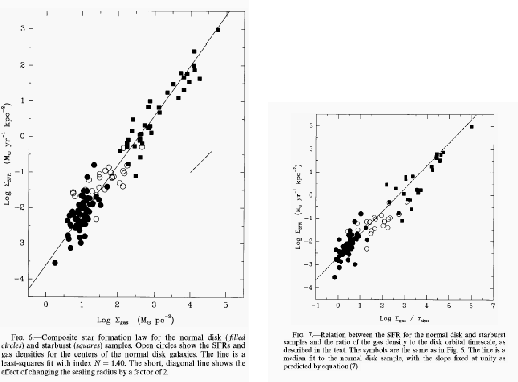The star formation rate is estimated by the intensity of H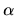emission (Kennicutt, Tamblyn, & Congdon 1994) as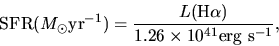(3.2)

which is used for normal galaxies. While in the starburst galaxies which show much larger star formation rate than the normal galaxies, FIR luminosity seems a better indicator of star formation rate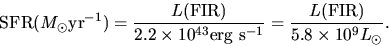(3.3)

Kennicutt (1998) summarized the relation between SFRs and the surface gas densities [Fig.3.1 (left)] for 61 normal spiral and 36 infrared-selected starburst galaxies. As seen in Figure 3.1, the star formation rate averaged over a galaxy (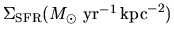), which is called the global star formation rate, is well correlated to the average gas surface density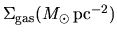. He gave the power of the global Schmidt law as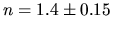. That is,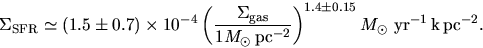(3.4)

The fact that the power is not far from 3/2 seems to be explained as follows: Star formation rate should be proportional to the gas density (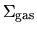) but it should also be inversely proportional to the time scale of forming stars in respective gas clouds, which is essentially the free-fall time scale. Remember the fact that the free-fall time given in equation (2.26) is proportional to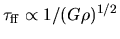. Therefore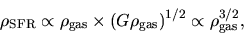(3.5)

where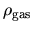and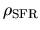are the volume densities of gas and star formation. He found another correlation between a quantity of gas surface density divided by the orbital period of galactic rotation and the star formation rate [Fig.3.1 (right)]. Although the actual slope is equal to 0.9 instead of 1, the correlation in Fig.3.1(right) is well expressed as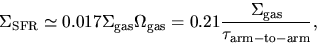(3.6)

where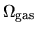represents the angular speed of galactic rotation. This means that 21 % of the gas mass is processed to form stars per orbit. These two correlations [eqs. (3.4) and (3.6)] implicitly ask another relation of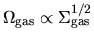.Next: Local Star Formation Rate Up: Schmidt Law Previous: Schmidt Law   Contents
Kohji Tomisaka 2007-07-08